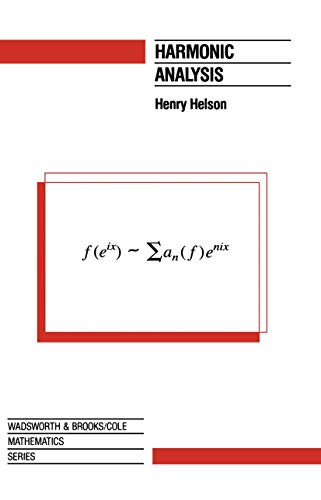# Harmonic Analysis by Henry Helson

Out of Stock
\$80.99
inc. GST
To be sure there is a big gap between learning the Banach-Steinhaus theorem, for example, and applying it to a real problem. It is a natural objective, because integration theory and functional analysis to a great extent developed in response to the problems of Fourier series!
Only 0 left

## Harmonic Analysis Summary

### Harmonic Analysis by Henry Helson

The reader is assumed to know the elementary part of complex funCtion theory, general topology, integration, and linear spaces. All the needed information is contained in a usual first-year graduate course on analysis. These prerequisites are modest but essential. To be sure there is a big gap between learning the Banach-Steinhaus theorem, for example, and applying it to a real problem. Filling that gap is one of the objectives of this book. It is a natural objective, because integration theory and functional analysis to a great extent developed in response to the problems of Fourier series! The exposition has been condensed somewhat by relegating proofs of some technical points to the problem sets. Other problems give results that are needed in subsequent sections; and many problems simply present interesting results of the subject that are not otherwise covered. Problems range in difficulty from very simple to very hard. The system of numeration is simple: Sec. 3. 2 is the second section of Chapter 3. The second section of the current chapter is Sec. 2. Formula (3. 2) is the second formula of Sec. 3, of the current chapter unless otherwise mentioned. With pleasure I record the debt to my notes from a course on Real Variables given by R. Salem in 1945. I wish to thank R. Fefferman, Y. Katznelson, and A. 6 Cairbre for sympathetic criti cism of the manuscript. Mr. Carl Harris of the Addison-Wesley Publishing Company has been most helpful in bringing the book to publication.

### Why buy from World of BooksOur excellent value books literally don't cost the earthFree delivery in AustraliaEvery used book bought is one saved from landfill

1 Fourier Series and Integrals.- 1.1. Definitions and easy results.- 1.2. The Fourier transform.- 1.3. Convolution; approximate identities; Fejer's theorem.- 1.4. Unicity theorem; Parseval relation; Fourier-Stieltjes coefficients.- 1.5. The classical kernels.- 1.6. Summability; metric theorems.- 1.7. Pointwise summability.- 1.8. Positive definite sequences; Herglotz' theorem.- 1.9. The inequality of Hausdorff and Young.- 1.10. Multiple Fourier series; Minkowski's theorem.- 1.11. Measures with bounded powers; homomorphisms of l1.- 2. The Fourier Integral.- 2.1. Introduction.- 2.2. Kernels on R.- 2.3. The Plancherel theorem.- 2.4. Another convergence theorem; the Poisson summation formula.- *2.5. Finite cyclic groups; Gaussian sums.- * Starred sections present material that is less fundamental..- 3. Hardy Spaces.- 3.1. Hp(T).- 3.2. Invariant subspaces, factoring, proof of the theorem of F. and M. Riesz.- 3.3. Theorems of Beurling and Szegoe.- 3.4. Structure of inner functions.- 3.5. Theorem of Hardy and Littlewood; Hilbert's inequality.- 3.6. Hardy spaces on the line.- 4. Conjugate Functions.- 4.1. Conjugate series and functions.- 4.2. Theorems of Kolmogorov and Zygmund.- 4.3. Theorems of M. Riesz and Zygmund.- 4.4. The conjugate function as a singular integral.- 4.5. The Hilbert transform.- 4.6. Maximal functions.- 4.7. Rademacher functions; absolute Fourier multipliers.- 5. Translation.- 5.1. Theorems of Wiener and Beurling; the Titchmarsh convolution theorem.- 5.2. The Tauberian theorem.- 5.3. Spectral sets of bounded functions.- *5.4. A theorem of Szegoe; theorem of Gru?ewska and Rajchman; idempotent measures.- 6. Distribution.- 6.1. Equidistribution of sequences.- 6.2. Distribution of (nku).- 6.3. Dynamical systems; (k2u).- Appendix. Integration by parts.- Bibliographic Notes.

GOR010173414
Harmonic Analysis by Henry Helson
Henry Helson
The Wadsworth & Brooks/Cole Mathematics Series
Used - Very Good
Paperback